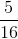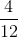## Example Questions

### Example Question #1 : Decimals

When 5/11 is written as a decimal, what is the 100th digit to the right of the decimal point?

1

2

5

0

4

5

Explanation:

When 5 is divided by 11, the decimal is 0.45 repeating, with a 5 in the hundreths place. The key here is to recognize that 100 is an even number, and the 5 in 0.45 is two places to the right of the decimal point (2 also being an even number).

### Example Question #2 : Decimals

Find  the sum of the following: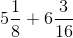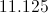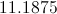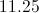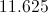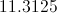Explanation:

To get the decimal from a fraction, divide the numerator by the denominator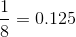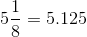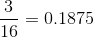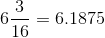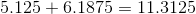### Example Question #3 : Decimals

Find the sum to the nearest hundredth: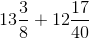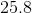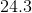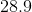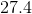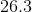Explanation:

1. Convert the fractions to decimals by division: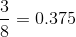2. Add the corresponding whole numbers to the decimals: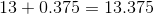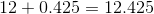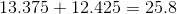### Example Question #1 : How To Find The Decimal Equivalent Of A Fraction

If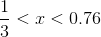can equal which of the following?None of the other answer choices are correct